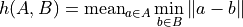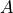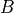# Mean Distance Between All Points on Two Curves#

## Synopsis#

Compute the mean distance between all points of two curves.

## Code#

### C++#

#include "itkImage.h"
#include "itkContourMeanDistanceImageFilter.h"

#ifdef ENABLE_QUICKVIEW
#  include "QuickView.h"
#endif

template <typename TImage>
static void
CreateImage1(TImage * const);

template <typename TImage>
static void
CreateImage2(TImage * const);

int
main()
{
using ImageType = itk::Image<unsigned char, 2>;

auto image1 = ImageType::New();
CreateImage1(image1.GetPointer());

auto image2 = ImageType::New();
CreateImage2(image2.GetPointer());

using ContourMeanDistanceImageFilterType = itk::ContourMeanDistanceImageFilter<ImageType, ImageType>;

ContourMeanDistanceImageFilterType::Pointer contourMeanDistanceImageFilter =
ContourMeanDistanceImageFilterType::New();
contourMeanDistanceImageFilter->SetInput1(image1);
contourMeanDistanceImageFilter->SetInput2(image2);
contourMeanDistanceImageFilter->Update();

std::cout << "Mean distance: " << contourMeanDistanceImageFilter->GetMeanDistance() << std::endl;

#ifdef ENABLE_QUICKVIEW
QuickView viewer;
viewer.Visualize();
#endif
return EXIT_SUCCESS;
}

template <typename TImage>
void
CreateImage1(TImage * const image)
{
// Create an image bigger than the input image and that has dimensions which are powers of two
typename TImage::IndexType start = { { 0, 0 } };

typename TImage::SizeType size = { { 20, 20 } };

itk::ImageRegion<2> region(start, size);

image->SetRegions(region);
image->Allocate();
image->FillBuffer(0);

// Create a diagonal white line through the image
for (typename TImage::IndexValueType i = 0; i < 20; ++i)
{
for (typename TImage::IndexValueType j = 0; j < 20; ++j)
{
if (i == j)
{
itk::Index<2> pixel = { { i, j } };
image->SetPixel(pixel, 255);
}
}
}
}

template <typename TImage>
void
CreateImage2(TImage * const image)
{
typename TImage::IndexType start = { { 0, 0 } };

typename TImage::SizeType size = { { 20, 20 } };

itk::ImageRegion<2> region(start, size);

image->SetRegions(region);
image->Allocate();
image->FillBuffer(0);

// Create a vertical line of white pixels down the center of the image
for (typename TImage::IndexValueType i = 0; i < 20; ++i)
{
for (typename TImage::IndexValueType j = 0; j < 20; ++j)
{
if (i == 10)
{
itk::Index<2> pixel = { { i, j } };
image->SetPixel(pixel, 255);
}
}
}
}


## Classes demonstrated#

template<typename TInputImage1, typename TInputImage2>
class ContourMeanDistanceImageFilter : public itk::ImageToImageFilter<TInputImage1, TInputImage1>

Computes the Mean distance between the boundaries of non-zero regions of two images.

ContourMeanDistanceImageFilter computes the distance between the set non-zero pixels of two images using the following formula:whereis the dir ected Mean distance andandare respectively the set of non-zero pixels in the first and second input images.

In particular, this filter uses the ContourDirectedMeanImageFilter inside to compute the two directed distances and then select the largest of the two.

The Mean distance measures the degree of mismatch between two sets and behaves like a metric over the set of all closed bounded sets - with properties of identity, symmetry and triangle inequality.

This filter requires the largest possible region of the first image and the same corresponding region in the second image. It behaves as filter with two input and one output. Thus it can be inserted in a pipeline with other filters. The filter passes the first input through unmodified.

This filter is templated over the two input image type. It assume both image have the same number of dimensions.

See

ContourDirectedMeanDistanceImageFilter

Author

Teo Popa, ISIS Center, Georgetown University

ITK Sphinx Examples: